### Total Q2 2023 Investments: \$4,690,000Funded \$4.3 million, consisting of \$3.3 million of senior subordinated debt and \$1.0 million of preferred equity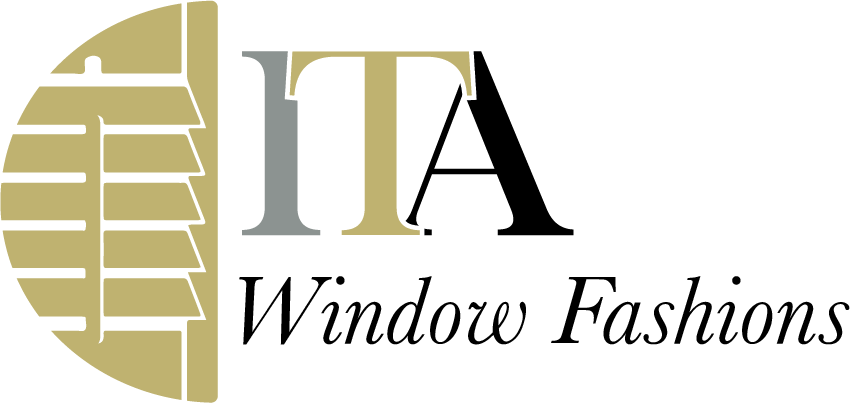Follow on debt investment of \$390,000

Total debt and equity investment in ITA had a fair value of \$4.0 million at quarter-end

## Total Q1 2023 Investments: \$5,550,000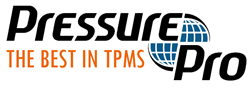Funded \$3.0 million in subordinated debt at 15% interest rate and with a 10% warrant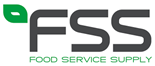Follow on debt investment of \$2.3 million in combination with an equity sale of \$210,000

Total debt and equity investment in FSS had a fair value of \$5.2 million at quarter-end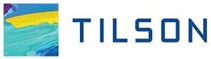Equity investment of \$250,000 in Tilson Technologies’ asset ownership affiliate SQF, LLC

Total debt and equity investment in Tilson and affiliated entities had a fair value of \$10.6 million at quarter-end

## Total Q4 2022 Investments: \$3,884,000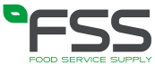Funded \$3.1 million, including \$2.5 million senior subordinate debt and \$600,000 preferred equityFollow on debt investment of \$590,000

Total debt and equity investment in SciAps had a fair value of \$5.2 million at year-endFollow on equity investment of \$194,000

Total debt and equity investment in Seyberts had a fair value of \$5.9 million at year-end

## Total Q3 2022 Investments: \$400,000Follow on equity investment of \$400,000

At quarter-end, total equity investment in ITA had a fair value of \$749,0000; Rand also has a \$3.5 million debt investment